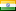### I need help for the python program for Mathematical Expressions

• Posted at : 1 month ago
• Post Similar Project
2500

Budget
24
Proposals
330
Views
Awarded
Status
Skills Required

Posted By -

SSIndia
4.4
Projects Posted : 42
Projects Paid : 18
Services Purchased : 0
Total Spent :
307
Feedbacks : 94 %

#### Project Details hide (-)

below is the example for doing the python codes based on the maths ..

->We will develop a data structure to represent arithmetic expressions containing variables, such as 3+4 or 2+x∗(1−y).
What is an expression? An expression consists of one of these:
1. A number
2. A variable
3. If e1 and e2 are expressions, then e1+e2, e1−e2, e1∗e2, and e1/e2 are also expressions.

Formally, the set of expressions is the least set constructed according to the rules above.
Thus, an expression can be either a constant, representing numbers
and variables, or a composite expression, consisting of an operator, a
left expression, and a right expression

There are (at least) two ways of representing expressions. The
simplest way is to represent expressions as trees, and define operations
on them.
The more sophisticated way consists in representing expressions via
classes: there will be one class for variable and constants, and one
class representing composite expressions; both of these classes will be sub-classes of a generic "expression" class.

i will share the complete requirement once you are able to understand the mathematical expressions